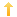## PowerBuilder – Back Tab to Last Field in DataWindow

Posted on Tuesday, March 23rd, 2021 at 7:11 pm in

An annoyance in a PB window with a datawindow control on it is if you back tab to the dw, your cursor is positioned to the first field and not the last field. The following technique can eliminate that and make your application a bit more user friendly.

In the Getfocus event of the datawindow:

``````long ll_col = 1
long ll_pos, ll_x, ll_y, ll_tab
long ll_curr_x, ll_curr_y
string ls_list, ls_piece, ls_column, ls_setting

// user back tabbed to get here
IF Keydown(keyshift!) AND Keydown(keytab!) THEN
// objects on datawindow
ls_list = THIS.object.datawindow.objects + '~t'
DO WHILE ls_list <> ''
ll_pos = POS(ls_list, '~t')
IF ll_pos > 0 THEN
ls_piece = MID(ls_list, 1, ll_pos - 1)
ls_list = TRIM(MID(ls_list, ll_pos + 1))
IF TRIM(ls_piece) <> '' THEN
ls_setting = THIS.Describe(ls_piece + '.Type')
IF UPPER(ls_setting) = 'COLUMN' THEN // only look at columns
ls_setting = THIS.Describe(ls_piece + '.Visible')
//check for visible only
IF ls_setting = "1"	THEN
ls_setting = THIS.Describe(ls_piece + '.X')
ll_x = long(ls_setting)
ls_setting = THIS.Describe(ls_piece + '.Y')
ll_y = long(ls_setting)
ls_setting = THIS.Describe(ls_piece + '.TabSequence')
ll_tab = long(ls_setting) //check tab sequence
IF ll_tab > 0 AND ((ll_y = ll_curr_y AND ll_x > ll_curr_x) OR (ll_y > ll_curr_y)) THEN
ll_curr_x = ll_x
ll_curr_y = ll_y
ls_column = ls_piece
END IF
END IF
END IF
END IF
END IF
LOOP
IF TRIM(ls_column) <> '' THEN
THIS.setrow(long(THIS.Object.DataWindow.LastRowOnPage))
THIS.setcolumn(ls_column)
END IF
END IF``````

On any given datawindow (if you put this in an ancestor) you need to make sure that any columns you place ‘out of view’ are either invisible or have a tab sequence of zero.

You might also be interested in
Tags: